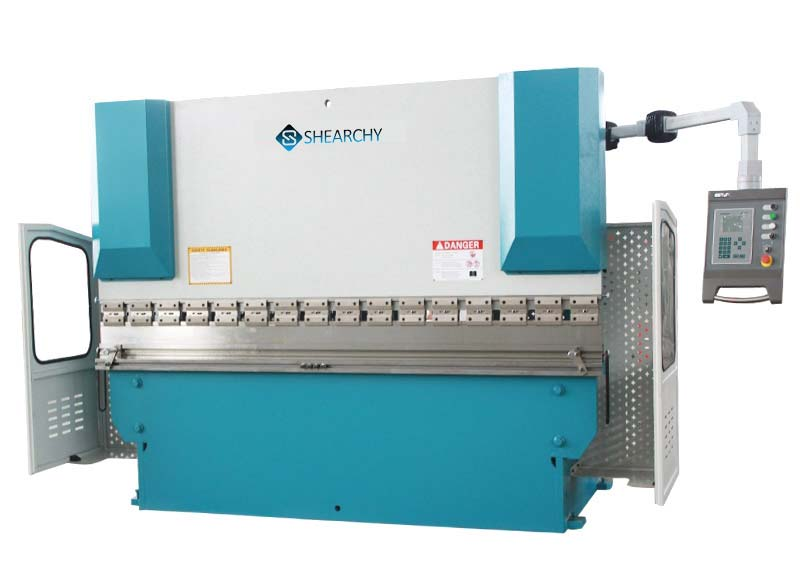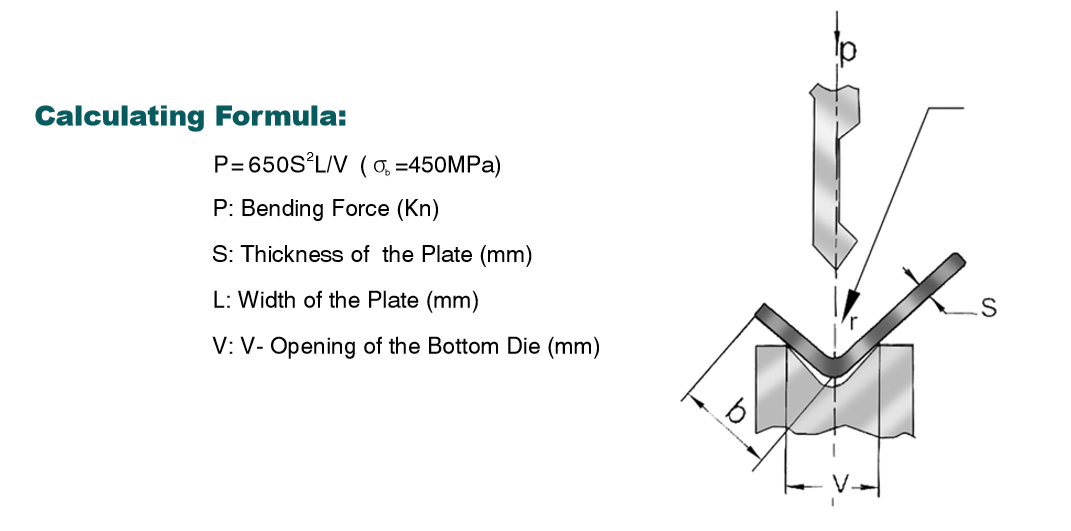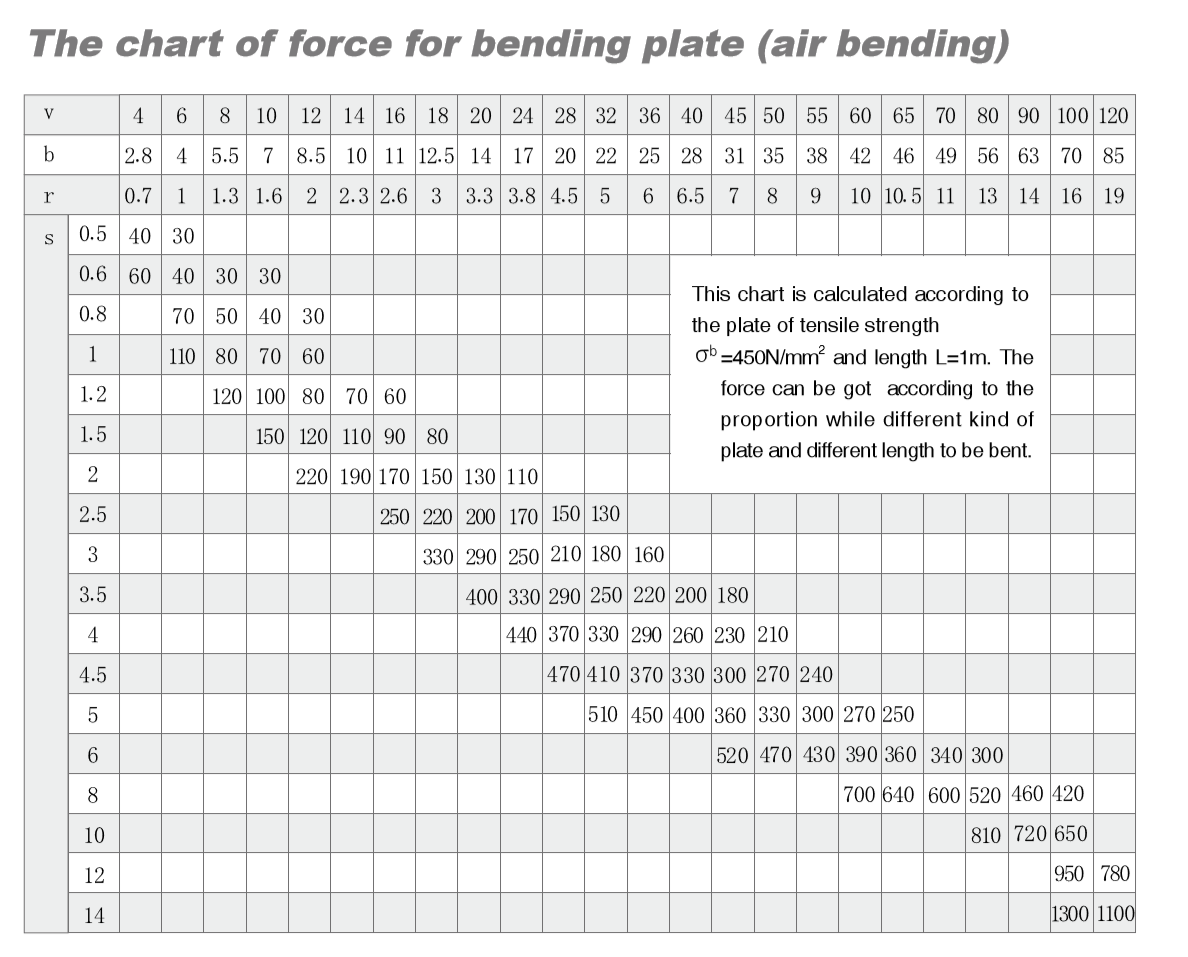## How to calculate Press Brake Tonnage?

During the bending process, the force between the upper and lower dies is applied to the material to cause plastic deformation of the material. Working tonnage refers to the bending pressure at the time of bending. The factors influencing the working tonnage are: bending radius, bending method, mold ratio, elbow length, thickness and strength of bending plate material, as shown in Figure 1. Usually, the working tonnage can be selected as shown in the table below and set in the machining parameters. 1(Parameters.1 Press Brake dies and tonnage formula, provided by Wuxi Shenchong Forging Machine Co.,Ltd)

### Usually When people calculate the Press Brake Tonnage, they can follow below press brake tonnage chart.

1. The value in the chart is the bending pressure when the length of the sheet is one meter: Example: S=4mm L=1000mm V=32mm Check the table P=330kN
2. This table is calculated based on the material with the strength σb=450N/mm2. When bending other materials, the bending pressure is the product of the data in the table and the following coefficient; Bronze (soft): 0.5; Stainless steel: 1.5; aluminum (soft): 0.5 ; chrome molybdenum steel: 2.
3. Approximate calculation formula for bending pressure: P=650s2L/1000v, the unit of each parameter P——kN S——mm L——mm V——mm(Parameters.2 Press Brake Tonnage Chart, provided by Wuxi Shenchong Forging Machine Co.,Ltd)

### Note: The relationship between the tonnage of the bending machine and the MPa on the pressure gauge:

“MPa” is the unit of pressure. The pressure on the area of ​​one square meter is one Pascal. The pressure generated is one Pascal [1Pa=1N/(M× M)]. 1 MPa = 1,000,000 Pa.

The kilogram force is the unit of force: 1 kg force = 9.8 Newtons. This is the physical quantity of two different concepts, and it is impossible to say “1 MPa is equal to how many kilograms of force”. But they have a certain relationship with each other: to produce a pressure of “1 MPa”, it needs to be on an area of ​​1 square centimeter. The applied pressure is about 10 kg.

### How to choose the bending machine suitable for sheet bending.

The calculation formula of nominal pressure: P=650S*S*L/v

• P = Nominal pressure KN
• S = thickness mm
• L = bending plate length m
• V = notch width mm Where V is 8-10 times the thickness of the plate.

In general, we have two ways to calculate the press brake tonnage, one is use press brake tonnage chart and another is to use formula. Example: S=4mm L=3000mm V=32mm Check the table P=330kN, the total tonnage is 330kN x 3m = 990kN = 100Ton. By using the Formula, the tonnage should be P = 650 x 4 x 4x 3/ (8 x 4) =100 Ton. If the material is stainless steel, the tonnage is 100Ton x 1.5 =150 Ton.